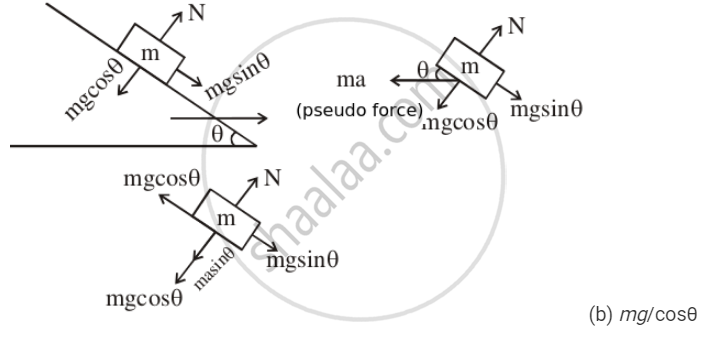# P a Block of Mass M is Placed on a Smooth Wedge of Inclination θ. the Whole System is Accelerated Horizontally So that the Block Does Not Slip on the Wedge. the - Physics

MCQ

A block of mass m is placed on a smooth wedge of inclination θ. The whole system is accelerated horizontally so that the block does not slip on the wedge. The force exerted by the wedge on the block has a magnitude.

• mg

• mg/cosθ

• mg cosθ

• mg tanθ

#### SolutionFree-body Diagram of the Small Block of Mass 'm'
The block is at equilibrium w.r.t. to wedge. Therefore,
mg sinθ = ma cosθ
⇒ a = gtanθ
Normal reaction on the block is
N = mg cosθ + ma sinθ
Putting the value of a, we get:
N = mg cosθ + mg tanθsinθ
$N = mg\cos\theta + mg\frac{\sin\theta}{\cos\theta}\sin\ \theta N=\frac{mg}{\cos\theta}$

Concept: Newton’s Second Law of Motion
Is there an error in this question or solution?

#### APPEARS IN

HC Verma Class 11, Class 12 Concepts of Physics Vol. 1
Chapter 5 Newton's Laws of Motion
MCQ | Q 6 | Page 77